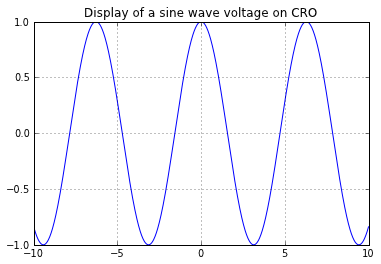# Chapter 14 :Electronic Instruments¶

### Example 14.1 Page no.443¶

In :
Rm=100.0                   #Ohms,  coil resistance
Is=100*10**(-6 )      #A current sensivity
Vr=100.0                     #V,  voltage

#Calculation
Rtotal=Vr/Is             #series resistance
#Result
print " The Series Resistance to Convert given dArsonval movement into a Voltmeter is, Rs =  ",Rs/10**3,"Kohm"

 The Series Resistance to Convert given dArsonval movement into a Voltmeter is, Rs =   999.9 Kohm


### Example 14.2 Page no. 445¶

In :
Rm=100.0                        #. meter resistance             #Ohms
CS=100*10**(-6)                 #A. current sensivity
Imax=10*10**(-3)                #A. maximum current

#Calculation
Ish=Imax-CS
Rsh=Rm*CS/Ish
# Result
print " The Value of Shunt Resistance is, Rsh =  ",round(Rsh,6),"ohm"

 The Value of Shunt Resistance is, Rsh =   1.010101 ohm


### Example 14.3 Page no.446¶

In :
CS=100*10**(-6)                              #A, current source
R=100.0                                               #Ohms, resistance
Rm=900.0                                            #Ohms, resistance of a meter

#(a)
#Calculation
Imax1=1*10**(-3)                             #A, maximum current
Rsh=CS*R/(Imax1-CS)                    #ohm, shunt path resistance
Rm1=Rm                                            #ohm,  meter branch resistance
Ish1=Imax1-CS                                 #A  shunt path current
Rsh1=Rm1*CS/Ish1                        #ohm shunt path resistance

#(b)
#Calculation
Imax2=0.01                              #A, maximum current
Ish2=Imax2-CS                                #A, shunt path current
R1=(R*Ish2-Rm*CS)/(Ish2+CS)    #ohm, resistance in branch 1

#(c)
#Calculation
Imax3=100*10**(-3)                        #A. maximum current
Ish3=Imax3-CS                                 #A, shunt path current
R2=((R-R1)*Ish3-Rm*CS)/(Ish3-CS)                        #ohm, resistance in branch 2

#(d)
#Calculation
Imax4=500*10**(-3)                       #A  , maximum current
Ish4=Imax4-CS                                #A, shunt path current
R3=((R-R1-R2)*Ish4-Rm*CS)/(Ish4-CS)                 #ohm, resistance in branch 3

#(e)
#Calculation
Imax5=1                                              #A
Ish5=Imax5-CS                                  #A, shunt path current
R4=((R-R1-R2-R3)*Ish5-Rm*CS)/(Ish5-CS)            #ohm, resistance in branch 4
R5=R-R1-R2-R3-R4                                                      #ohm, resistance in branch 2

# Result
print " Shunt Resistance  =",round(Rsh,6),"ohm"
print " For Range switch at 1 mA  , Rsh1 ",Rsh1,"ohm"
print " For Range switch at 10 mA , R1 =   ",R1,"ohm"
print " For Range switch at 100 mA, R2 =   ",round(R2,0),"ohm"
print " For Range switch at 500 mA, R3 =   ",round(R3,1),"ohm"
print " For Range switch at 1 A   , R4 =   ",round(R4,1),"ohm"
print "  R5 =   ",round(R5,1),"ohm"

 Shunt Resistance  = 11.111111 ohm
For Range switch at 1 mA  , Rsh1  100.0 ohm
For Range switch at 10 mA , R1 =    90.0 ohm
For Range switch at 100 mA, R2 =    9.0 ohm
For Range switch at 500 mA, R3 =    0.7 ohm
For Range switch at 1 A   , R4 =    0.1 ohm
R5 =    0.1 ohm


### Example 14.4 Page no.469¶

In :
DS=5                         #V/cm, Deflection Sensitivity
l=10                           #cm, Trace Length

#Calculation
import math
Vp=DS*l
Vm=Vp/2
V=Vm/math.sqrt(2)
# Result
print " The RMS AC Voltage is=",round(V,3),"V"

 The RMS AC Voltage is= 17.678 V

In :
%matplotlib inline

Welcome to pylab, a matplotlib-based Python environment [backend: module://IPython.zmq.pylab.backend_inline].


### Example 14.5 Page no. 471¶

In :
import numpy
#Given Circuit Data
Am=3.5                                        #V, Amplitude
tb=0.1*10**(-3)                          #seconds
TP=4                                            #Time Period
x=linspace(-10,10,1000)
plt.grid()
plot(x,cos(x))
title('Display of a sine wave voltage on CRO ')
show()
#Calculation
import math
Vm=2*Am
V=Vm/math.sqrt(2)
T=TP*tb
f=1/T

# Result
print "The Magnitude of Wave Voltage, ",round(V,2),"V"
print " The Frequency of Wave Voltage, f = ",f/10**3,"KHz"The Magnitude of Wave Voltage,  4.95 V
The Frequency of Wave Voltage, f =  2.5 KHz